# Clifford semi-group

(diff) ← Older revision | Latest revision (diff) | Newer revision → (diff)

completely-regular semi-group

A semi-group in which every element is a group element, that is, lies in some subgroup. An element of a semi-group is a group element if and only if it is completely regular (see Regular element). A semi-groupis a Clifford semi-group if and only if either of the following conditions holds: 1) For every,; or 2) each one-sided idealofis isolated (or semi-prime), that is, ifthen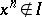for all natural numbers.

Together with inverse semi-groups (cf. Inversion semi-group), Clifford semi-groups represent one of the most important types of regular semi-groups. Their study was begun in the fundamental paper  of A.H. Clifford. Every Clifford semi-group has a (unique) decomposition into groups, the classes of which are exactly the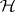-classes (cf. Green equivalence relations). Such a decomposition is not necessarily a band (see Band of semi-groups); conditions for this to be so are known (see ). The Green relations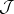and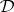on a Clifford semi-group coincide. Every completely-simple semi-group is a Clifford semi-group; a Clifford semi-group is completely simple if and only if it is simple (see Simple semi-group). Every Clifford semi-groupcan be decomposed into a semi-lattice of completely-simple semi-groups; this decomposition is unique, its components are just the-classes, and the corresponding quotient semi-lattice is isomorphic to the semi-lattice of principal ideals of. Conversely, every semi-group decomposable into a semi-lattice of completely-simple semi-groups is a Clifford semi-group.

The following conditions are equivalent for a Clifford semi-group: 1)is inverse; 2) every idempotent oflies in the centre, that is, it commutes with every element of; 3) every one-sided ideal ofis two-sided; 4) the Green relationsandoncoincide; 5)is a semi-lattice of groups; and 6)is a subdirect product of groups and groups with zero.

The decomposition of an arbitrary Clifford semi-group into a semi-lattice of completely-simple semi-groups defines its "global structure" . The multiplication law for elements within the components of this decomposition is given by Rees' theorem (see Completely-simple semi-group). Further investigations into Clifford semi-groups are to a large extent directed towards a clarification of their "fine structure" , that is, to determining multiplication laws of elements from different components. When the components are groups, that is, for inverse Clifford semi-groups, there is a constructive description in terms of a so-called sum of a direct spectrum of groups. Let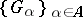be a family of pairwise disjoint groups, letbe a semi-lattice (see Idempotents, semi-group of) such that for every pair of elements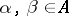such that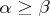, there is a homomorphism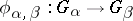such that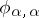is the identity automorphism for everyand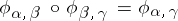whenever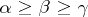. A productis defined on the union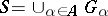by settingfor arbitraryand.

Thenbecomes an inverse Clifford semi-group. Conversely, every inverse Clifford semi-group can be obtained in this way.

The problem of the "fine structure" of Clifford semi-groups is in general extremely complicated, and to date (1987) there is no satisfactory solution to it. Certain very complex constructions, describing Clifford semi-groups in terms of completely-simple semi-groups, their translation hulls, semi-lattices, and mappings with special properties, are to be found in . Great progress has been achieved in the case of orthodox Clifford semi-groups (see Regular semi-group); such semi-groups are called orthogroups. There are a few clear, if somewhat cumbersome, constructions for them (see ). All the constructions mentioned generalize, in some way, the description of inverse Clifford semi-groups obtained in .

How to Cite This Entry:
Clifford semi-group. Encyclopedia of Mathematics. URL: http://encyclopediaofmath.org/index.php?title=Clifford_semi-group&oldid=14829
This article was adapted from an original article by L.N. Shevrin (originator), which appeared in Encyclopedia of Mathematics - ISBN 1402006098. See original article